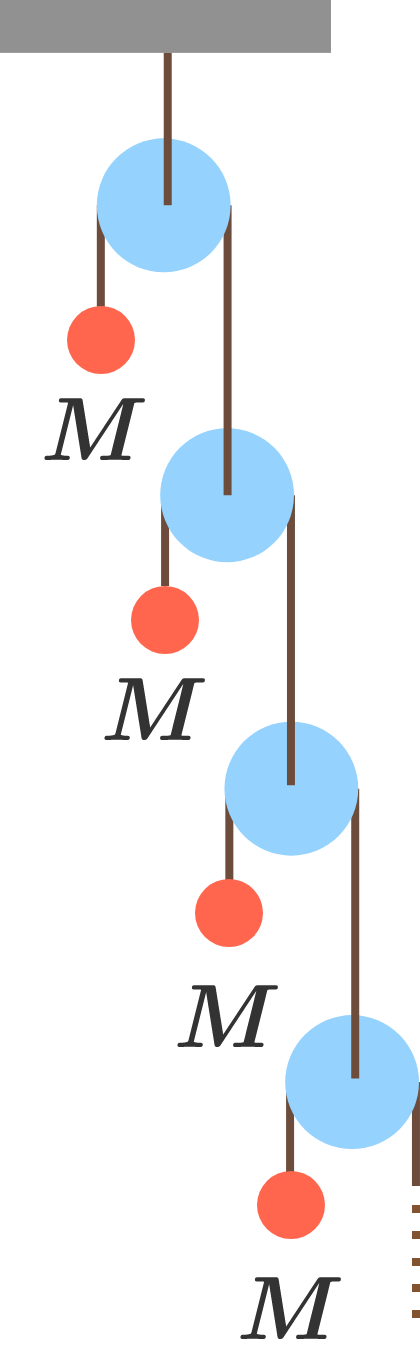Infinite Atwood’s machineThe infinite Atwood’s machine shown has a string passing over each pulley, with one end attached to a mass and the other end attached to another pulley.

The masses are held fixed and then released simultaneously, and the acceleration of the top mass is $\lambda g.$

What is $\lambda?$

Details and Assumptions:

• All the masses are equal to $M$.
• All the pulleys and strings are massless.
• $g$ is the acceleration due to gravity.
×